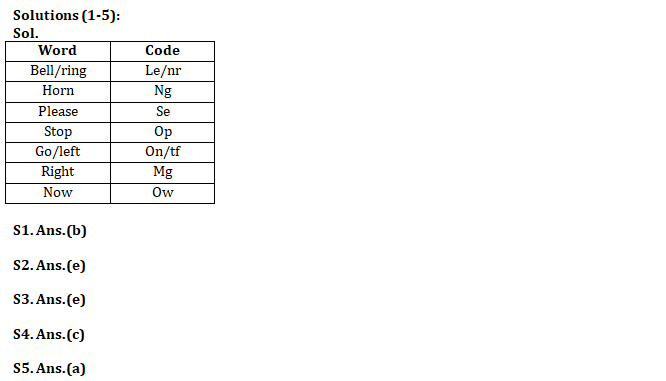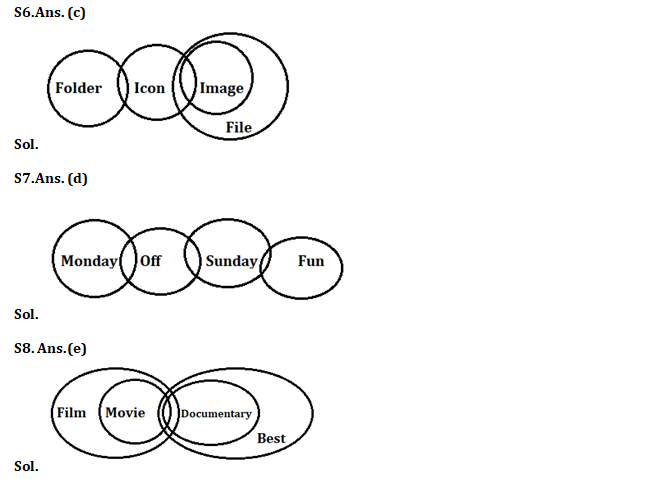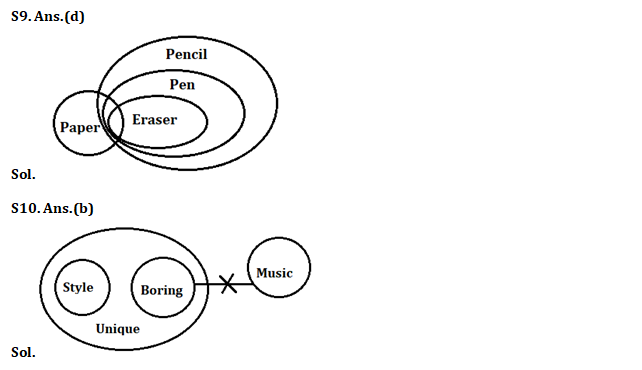Latest Banking jobs   »   Reasoning Ability Quiz For IBPS Clerk/PO...

# Reasoning Ability Quiz For IBPS Clerk/PO Prelims 2022- 4th September

Directions (1-5): Study the following information carefully and answer the questions given below:
In a certain code language:
‘Bell Ring Horn’ is written as ‘le ng nr’,
‘Stop Horn Please’ is written as ‘ng op se’,
‘Go right Left’ is written as ‘on mg tf’
‘Stop Right Now’ is written as ‘op mg ow’.

Q1. What is the code for ‘Please’ in the given code language?
(a) op
(b) se
(c) ng
(d) mg
(e) None of these

Q2. What is the code for ‘Right’ in the given code language?
(a) ng
(b) on
(c) tf
(d) ow
(e) None of these

Q3. What is the code for ‘left’ in the given code language?
(a) tf
(b) ow
(c) on
(d) mg
(e) Can’t be determined

Q4. What may be the code for ‘Stop Horn Ring’ in the given code language?
(a) mg ng nr
(b) mg op nr
(c) op ng nr
(d) tfow op
(e) None of these

Q5. What may be the code for ‘take left’?
(a) km on
(b) km mg
(c) mg tf
(d) ow op
(e) None of these

Directions (6-10): In each of the questions below, some statements are given followed by some Conclusions. You have to take the given statements to be true even, if they seem to be at variance from commonly known facts. Read all the conclusions and then decide which of the given conclusions logically follows from the given statements disregarding commonly known facts.

Q6. Statements:Some Icon are Image
All Image are File
Some Folder are Icon
Conclusions:I: Some Folder are not File
II: All Folder are File

(a) If only conclusion I follows.
(b) If only conclusion II follows.
(c) If either conclusion I or II follows.
(d) If neither conclusion I nor II follows.
(e) If both conclusions I and II follow.

Q7. Statements:Some Monday are Off
Some Off are Sunday
Some Sunday are Fun
Conclusions:I: Some Fun are not Monday
II: All Monday are Fun

(a) If only conclusion I follows.
(b) If only conclusion II follows.
(c) If either conclusion I or II follows.
(d) If neither conclusion I nor II follows.
(e) If both conclusions I and II follow.

Q8. Statements: All Movie are Film
Some movie are Documentary
All Documentary are Best
Conclusions: I. Some film are best
II. Some Documentary are Film.

(a) If only conclusion I follows.
(b) If only conclusion II follows.
(c) If either conclusion I or II follows.
(d) If neither conclusion I nor II follows.
(e) If both conclusions I and II follow.

Q9. Statements:Some paper is Eraser
All Eraser is pen
All Pen are Pencil
Conclusions: I. Some Paper are not Pencil
II. Some Paper are Pen is a possibility.

(a) If only conclusion I follows.
(b) If only conclusion II follows.
(c) If either conclusion I or II follows.
(d) If neither conclusion I nor II follows.
(e) If both conclusions I and II follow.

Q10. Statements: All Style are Unique
No Music is Boring
All Boring are Unique
Conclusions: I. Some style are not Music.
II. Some Unique are not Music

(a) If only conclusion I follows.
(b) If only conclusion II follows.
(c) If either conclusion I or II follows.
(d) If neither conclusion I nor II follows.
(e) If both conclusions I and II follow.

Directions (11-15): Following questions are based on the five three-digit numbers given below.

123 320 287 424 521

Q11. If all the digits in each of the numbers are arranged in ascending order within the number, then, which of the following number will become the highest in the new arrangement of numbers?
(a) 123
(b) 320
(c) 287
(d) 424
(e) 521

Q12. If all the numbers are arranged in descending order from left to right then, which of the following will be the product of 3rd digit of 2nd number from left and 3rd digit of 2nd number from right in new arrangement?
(a) 26
(b) 24
(c) 28
(d) 20
(e) 30

Q13. What will be the resultant when 3rd digit of the highest number is multiplied with the 2nd digit of the lowest number?
(a) 2
(b) 6
(c) 8
(d) 16
(e) 12

Q14. If the positions of the second and the third digits of each of the numbers are interchanged then, how many odd numbers will be formed?
(a) None
(b) One
(c) Two
(d) Three
(e) Four

Q15. If one is subtracted from each of the numbers then, how many numbers thus formed will be divisible by three?
(a) None
(b) One
(c) Two
(d) Three
(e) Four

SolutionsS11. Ans. (c)

S12. Ans. (c)

S13. Ans. (a)

S14. Ans. (a)

S15. Ans. (b)#### Congratulations!Download Hindu Review of October 2021: Free PDF